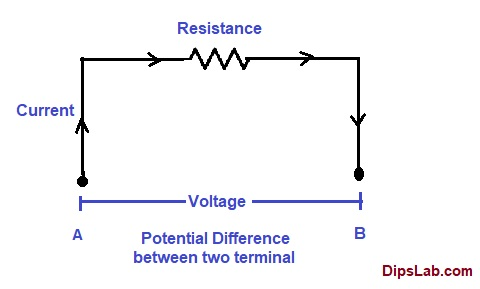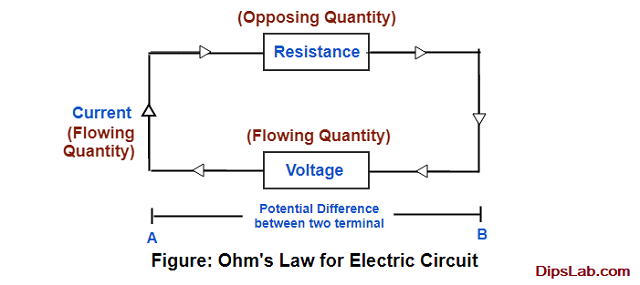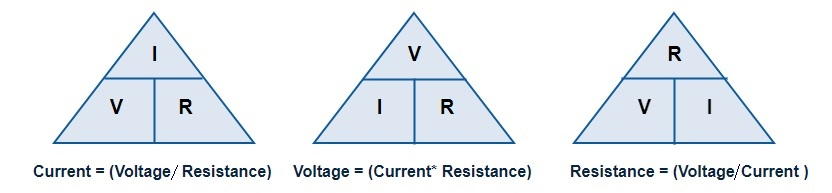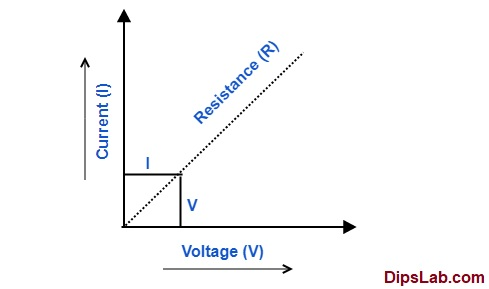# Ohm’s Law Statement | Formula, Limitations and ApplicationsToday, I am describing ohm’s law of electricity. It is one of the basic fundamental electrical laws.

Ohm’s law is related to the three basic electrical quantities such as electric current, potential difference (or voltage), and resistance.

To learn about history, who did discover the ohm’s law?

By its name, it’s not really difficult to guess. The ohm’s law was discovered by German physicist scientist Dr. Georg Simon Ohm in 1927.

### What is Ohm’s Law?

Ohm’s law is important and essential in every electrical circuit, especially for the Direct Current (DC) circuit.

This law is applicable in case of a good conductor.

Ohm’s Law Statement:

Ohm’s law is stated as,

An electric current (I) passes through the conductor are directly proportional to the applied voltage (V) between the two terminal of conductor, if physical conditions remain constant.

This law is obeyed if the physical condition does not change.

Let’s understand some basics.

How does the current flow in the circuit?

The number of free electrons randomly flows through a conductor. These free electrons collide with each other. The collision process emits its energy in the form of heat.

That generates potential energy or voltage difference between the two terminal (A and B) of the conductor. Because of the voltage difference, electrons flow from one terminal to another terminal.

Here, resistance helps to flow and produce opposition for the collision of electrons. Thus, resistance protects the conductor from the heating effect.Ohm’s Law for Electricity Analogy

So, resistance is the most important factor for limiting current circulation and protecting the electrical system.

I have explained resistance in detail.

Representation:

`Electric current (I) ∝ Potential Difference (V)`

Formula:

`[Potential Difference (V) / Electric current (I)] = Constant`

Here, resistance (R) is the constant factor.

`[Potential Difference (V) / Electric current (I)] = Resistance (R)`

In the general term,

The forcing quantities (V) is directly proportional to the flowing qunantities if the opposing quantities (R) is constant.

From the following image, you can easily understand.This means, if the voltage increases, current increases in the electrical circuit.

Representation:

` Forcing quantity (V)  ∝ Flowing quantity (I)`
`Forcing quantity (V) ∝ Opposing quantity (R)`

This is the simple explanation of Ohm’s Law.

Ohm’s law is basic for Kirchhoff Current Laws and Kirchhoff Voltage Laws.

### Ohm’s Law Calculator

You can calculate any one unknown electric quantities  (from electric current, potential difference, and resistance) if the other two are given.Triangle Method for Ohm’s law

I have created online calculators to calculate these values. You can use them directly.

1. Electric Current

It is expressed in Ampere (A).

Related Read: Electric Current in Detail

2. Voltage or Potential Difference

Potential Difference or Voltage is expressed in Volt (V).

3. Resistance

Resistance is expressed in ohm (Ω).

The resistor is physical equipment and it can have different resistance values. You can calculate the resistance value of the resistor using color codes.

### Is Ohm’s Law applicable for both AC and DC?

Ohm’s law is only applicable to direct current (DC).

It is not applicable to alternating current (AC) in the circuit. Alternating current (AC) continuously varies with time at a constant frequency (50Hz or 60 Hz).  This AC waveform occurs in the sinusoidal in nature.

Related Read: Alternating and Direct Current

### How is Ohm’s Law represented Graphically?

According to the principle of ohm’s law, an electric current (I) flow through a conductor is directly proportional to the applied voltage (V) and inversely proportional to the resistance (R).

It means that, when low current is flowing through the conductor, higher resistance occurs in the circuit and vice-versa.

In the graphical representation, we are explaining the relationship between electric current, voltage and resistance.

Here is plotting voltage on the x-axis and current on the y-axis of the graph.

The straight-line gives resistance.Characteristic of Electric Current (I) and Voltage (V)

### What are the Limitations of Ohm’s Law?

There are certain limitations of ohm’s law.

1. It is applicable for metallic conductors, not the non-metallic conductors.
2. In practical, when you apply ohm’s law, resistance should be constant.
3. Ohm’s law calculation becomes difficult in complicated circuits.
4. It does not apply to the nonlinear and unilateral elements. The law is used for linear elements and bilateral elements of the circuit. (I have explained different Electrical Circuit Elements.)

### What are the Applications of Ohm’s Law?

There are different applications of ohm’s law.

1. This law is used to determine the different electrical quantities such as current, voltage, resistance, power, conductance, etc.
2. There are many electrical appliances like electric heater, kettle operates based on the principle of ohm’s law.
3. It is useful for solving problems related to the equivalent series, parallel and series-parallel electric circuits.

This is all about ohm’s law statement, explanation, its limitations, and useful applications. If you any queries, you can comment below.

I am coming with another electrical tutorial, soon. Till then, stay tuned!

If You Appreciate What I Do Here On DipsLab, You Should Consider:

DipsLab is the fastest growing and most trusted community site for Electrical and Electronics Engineers. All the published articles are available FREELY to all.

If you like what you are reading, please consider buying me a coffee ( or 2 ) as a token of appreciation.

This will help me to continue my services and pay the bills.I’m thankful for your never-ending support.

### 5 thoughts on “Ohm’s Law Statement | Formula, Limitations and Applications”

1.Seriously, awesome. I like it. I am an Electrical engineer in R&d compliance. Your explanation is really outstanding.

•Thank you, Nirod Ranjan Samantaray!

2.It’s one of the best approaches I ever study to understand ohm’s law. Thank you so much for the explanation and made it an understandable way to everyone. It’s quite good.

•3.